Algebraic Equations Terms of Use    Contact Person: Donna Roberts1.
A recipe calls for three times as many blueberries
(b) as strawberries (s). Which choice represents this situation?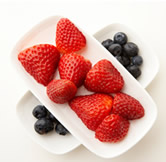Choose:
 s = 3b b = 3s sb s + b

2.
Express the total weight, (p), of a filled animal watering pail, if the pail weighs 12 pounds and the water weighs 8.3 pounds per gallon? [Let gallons = g.]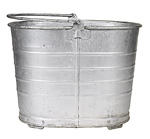Choose:
 p = (8.3)(12) p = 8.3g - 12 p = 8.3g + 12 p = 12g + 8.3

3.
Carl is thinking of a number. He states that twice the number decreased by six is twenty-four. Which choice can be used to find Carl's number?Choose:
 2n + 6 = 24 2(n - 6) = 24 2(n + 6) = 24 2n - 6 = 24

4.
A formula defines a value (v) to be one-half of the sum of three consecutive integers. Which choice represents this situation? [Let x = the first integer.]Choose:
 v = 0.5(3x) v = 0.5(x + x+1 + x+2) v = 0.5(x + 3) v = 0.5(x + x+2 + x+4)

5.
The "Bulbs On the Bay" holiday drive-through attraction charges \$12 per car plus \$1 for every individual, (p), in the car. Which choice represents the total cost (c) per car?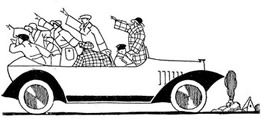Choose:
 c = p + 12 c = 12(p + 1) c = 12p + 1 c = 1•(12p)

6.
A cell phone charges \$65 for the phone and \$24 a month for the service. Which choice represents the cost (c) of the phone for the first m months?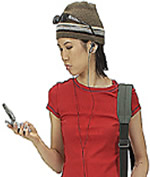Choose:
 c = 12m + 89 c = (24m + 65)/12 c = 24m - 65 c = 24m + 65

7.
At the mall, a soda costs \$1.95 (s), a burger costs \$3.20 (b), and chips cost \$2.10 (c).

Gelato is an Italian form of ice cream. A gelato booth sells small gelato cones for \$2.95 each (g).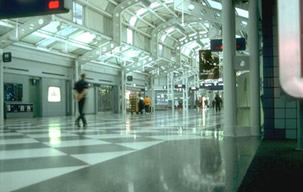a. Carlos buys a burger, a soda and chips for himself and each of his friends ( f ). Which choice represents his total cost (t), without tax ?
Choose:
 t = f (b + s + c) t = (f + 1)(b + s + c) t = f + 1(b + s + c) t = f (b + s + c)

b. Two of Carlos' friends do not want chips. Which choice will amend the answer to part a to reflect this change in the total cost?
Choose:
 t = f (b + s + c - 2) t = f (b + s + c) - 2c t = (f + 1)(b + s - 2c) t = (f + 1)(b + s + c) - 2c

c. Carlos' friend Amanda, says she will pay for the herself and each of the friends to have a gelato cone. Three of the friends do not want a cone. Which choice represents Amanda's total charge (ch), without tax?
Choose:
 ch = g(f - 2) ch = (f + 1)g - 3 ch = g(f - 3) ch = (f + 1)(g - 3)